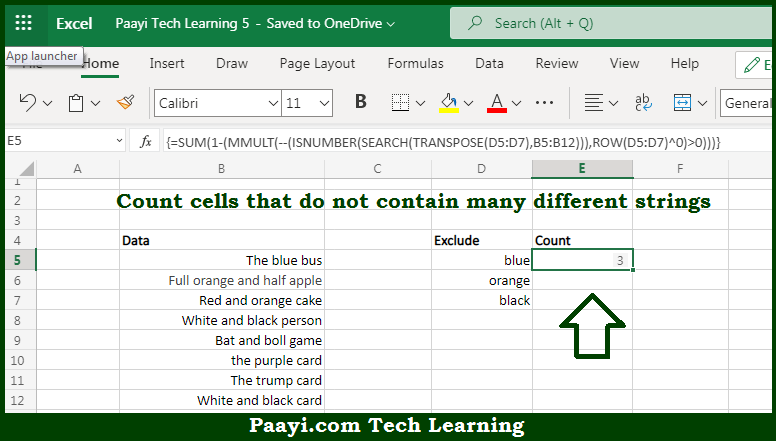# Learn How to COUNT Cells that Do Not Contain Many Strings in Microsoft Excel

Written by | 0 Comments | 583 Views

In this article, you will learn how to COUNT various things in Microsoft Excel using a single or combination of functions and its purpose in Microsoft Excel. You will also get to know how to COUNT cells that do not contain many strings.

Count Cells that Do Not Contain Many Strings in Microsoft Excel

The main purpose of this formula is to count cells that do not contain many different strings. Here we will learn how to count the range of cells not containing any kind of string/strings in Microsoft Excel.  That implies, with the help of a formula based on the  MMULT function you can able to count the number of cells in a range that does not contain many strings. So, with the help of this formula, you can able to count the number of cells that do not contain many strings.

General Formula to COUNT Cells that Do Not Contain Many Strings

{=SUM(1-(MMULT(--(ISNUMBER(SEARCH(TRANSPOSE(exclude),data))),ROW(exclude)^0)>0))}

The Explanation for the COUNT Cells that Do Not Contain Many StringsSo we know that with the help of the given formula above you can able to count cells that do not contain many different strings. Here we will learn how to count the range of cells not containing any kind of string/strings in Microsoft Excel. As we know that, the formula provided here, is complicated by the "contains" requirement.

In case you just need the formula to count cells that do not *equal* many things, you can use an easier formula based on the MATCH function. It should be noted that, if you have a limited number of strings to exclude, you can use the COUNTIFS function. So, with the help of this formula, you can able to count cells that do not contain many strings.